# Unequal probability outcomes#### Everything You Need in One Place

Homework problems? Exam preparation? Trying to grasp a concept or just brushing up the basics? Our extensive help & practice library have got you covered.#### Learn and Practice With Ease

Our proven video lessons ease you through problems quickly, and you get tonnes of friendly practice on questions that trip students up on tests and finals.#### Instant and Unlimited Help

Our personalized learning platform enables you to instantly find the exact walkthrough to your specific type of question. Activate unlimited help now!

0/3
##### Intros
###### Lessons
1. Introduction to Probability Outcomes for Coins, Dice, and Spinners:
2. What is probability?
3. Even chances vs. not even chances
4. Review on key probability terms
0/6
##### Examples
###### Lessons
1. Outcomes are the result of flipping coins, rolling dice, or turning a spinner's arrow
List all the possible outcomes. How many outcomes are there in total?
1. A spinner with 5 parts with the colors red, blue, or yellow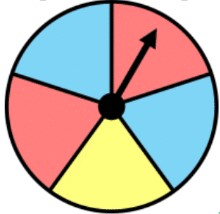2. A bag of 7 marbles with the colors blue, yellow, green or orange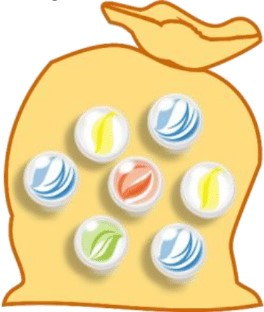2. Outcomes that are NOT equally as likely to occur
Write the probability in proper notation using:

$P$(event) = $\frac{number\,ways\,to\,get\,the\,outcome}{number\,total\,ways\,of\,getting\,outcomes}$
1. Spinning a spinner and landing on blue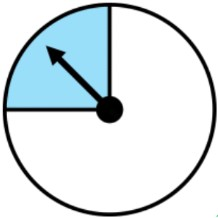2. Pulling out a green ball from a bag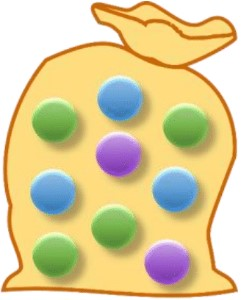3. Spinning a spinner and landing on the letter $G$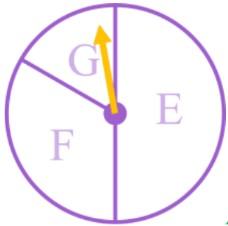4. Spinning a spinner and landing on red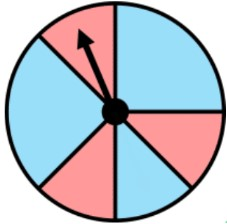###### Topic Notes

In this lesson, we will learn:

• In some probability scenarios, the outcomes are NOT all equally as likely to happen. Some outcomes will happen more often than others (have a greater chance of happening).
• How to write a probability fraction for events that have unequal outcomes

Notes:

• The outcomes for probability situation examples (coin toss, dice roll, equal parts spinner) have all had the same chance of happening. When all outcomes are just as likely (equally likely) to happen, there are even chances or equal chances of happening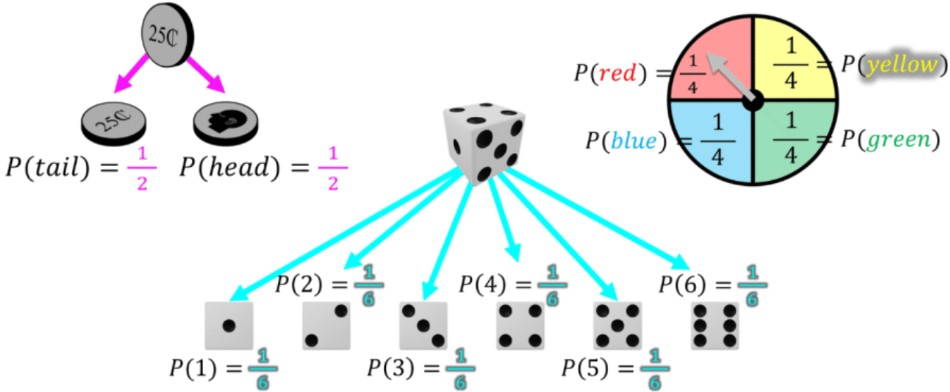• When all outcomes are just as likely (equally likely) to happen, it is called having even chances
• Probabilities can be compared across different situations. The outcomes of the coin are more likely than the spinner, which are more likely than the outcomes of the die ($\frac{1}{2}$ > $\frac{1}{4}$ > $\frac{1}{6}$ )

• It is also possible for the outcomes to NOT be equally as likely; there are unequal chances of happening

Ex. unequal vs. equal outcomes for a spinner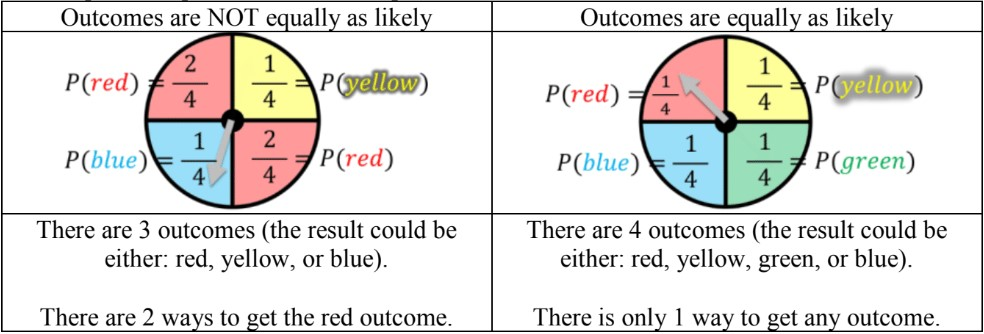• The probability for situations with unequal outcomes can be given as a fraction in this formula format:

$P$(event) = $\frac{number\,ways\,to\,get\,the\,outcome}{number\,total\,ways\,of\,getting\,outcomes}$